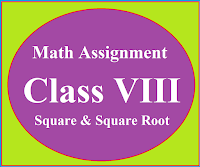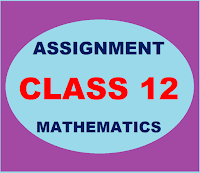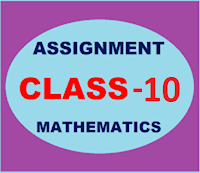## Posts

Showing posts from December, 2022

### Math Assignment Class VIII | Square & Square RootMath Assignment  Class VIII | Square & Square Root Download or Print free  assignment with answer key  for   Class  8 Squares and  Square Roots.   Important and extra questions that cover all topics of square and square root and is useful and helpful for the students. Math Assignment  Class VIII | Square & Square Root LEVEL -1

### CBSE Assignments class 12 MathematicsCBSE Assignments class 12 Mathematics Chapter-wise mathematics assignment for class 12. Important and useful extra questions strictly according to the CBSE syllabus and pattern with answer key CBSE Mathematics is a very good platform for the students and is contain the assignments for the students from 9 th  to 12 th  standard.  Here students can find very useful content which is very helpful to handle final examinations effectively.  For better understanding of the topic students should revise NCERT book with all examples and then start solving the chapter-wise assignments.  These assignments cover all the topics and are strictly according to the CBSE syllabus.  With the help of these assignments students can easily achieve the examination level and  can reach at the maximum height. Class 12 Mathematics    Assignment Case Study Based Question Chapter 1: Mathematics Assignment on Re lations & Functions Chapter 2:  Mathematics Assignment on  Inverse Trigonometric Functions Chap

### CBSE Assignments class 10 MathematicsAssignments Class 10 Mathematics Chapter-wise mathematics assignment for class 10. Important and useful extra questions strictly according to the CBSE syllabus and pattern with answer key CBSE Mathematics is a very good platform for the students and is contain the assignments for the students from 9 th to 12 th standard.  Here students can find very useful content which is very helpful to handle final examinations effectively.  For better understanding of the topic students should revise NCERT book with all examples and then start solving the chapter-wise assignments.  These assignments cover all the topics and are strictly according to the CBSE syllabus.  With the help of these assignments students can easily achieve the examination level and  can reach at the maximum height. Class 10 Mathematics   Assignment Case Study Based Questions Class X : Click Here Chapter 1: Mathematics Assignment on Real Numbers Chapter 2:  Mathematics Assignment Polynomial